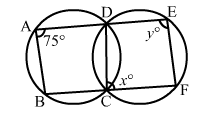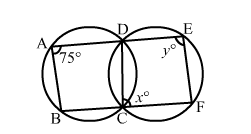# In the given figure, ∠BAD = 75°, ∠DCF = x°

Question:

In the given figure, BAD = 75°, ∠DCF = x° and ∠DEF = y°. Find the values of x and y.Solution:We know that if one side of a cyclic quadrilateral is produced, then the exterior angle is equal to the interior opposite angle.
i.e., ∠BAD = ∠DCF = 75°
⇒ ∠DCF = x  = 75°

Again, the sum of opposite angles in a cyclic quadrilateral is 180°.
Thus,
∠DCF + ∠DEF = 180°
75° + = 180°
⇒ y = (180° - 75°) = 105°

Hence, x = 75° and y = 105°